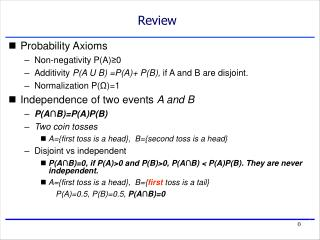Download PresentationReview

# Review - PowerPoint PPT PresentationDownload Presentation## Review

- - - - - - - - - - - - - - - - - - - - - - - - - - - E N D - - - - - - - - - - - - - - - - - - - - - - - - - - -
##### Presentation Transcript

1. Review • Probability Axioms • Non-negativity P(A)≥0 • Additivity P(A U B) =P(A)+ P(B), if A and B are disjoint. • Normalization P(Ω)=1 • Independence of two events A and B • P(A∩B)=P(A)P(B) • Two coin tosses • A={first toss is a head}, B={second toss is a head} • Disjoint vs independent • P(A∩B)=0, if P(A)>0 and P(B)>0, P(A∩B) < P(A)P(B). They are never independent. • A={first toss is a head}, B={first toss is a tail} P(A)=0.5, P(B)=0.5, P(A∩B)=0

2. 1.3 Conditional Probability 1.4 Total Probability Theorem and Bayes’ Rule

3. Conditional Probability • A way to reason about the outcome given partial information • Example1 • To toss a fair coin 100 times, what’s the probability that the first toss was a head? • Fair coin 1/2 • To toss a fair coin 100 times, if 99 tails come up, what’s the probability that the first toss was a head? • Very small? • Example2 • A fair coin and an unfair coin (1/4 tail, 3/4 head) • The first toss is fair, if the outcome is a head, use the fair coin for the 2nd toss, if the outcome is a tail, use the unfair coin for the 2nd toss. • What’sthe probability that the 2nd toss was a tail? • ½x½ + ½x¼ = 0.375 • What’s the probability that the 2nd toss was a tail if we know that the first toss was a tail? • 1/4

4. Conditional Probability • Definition of a conditional probability • The probability of event A given event B (P(B)>0 ) • P(A|B)=P(A) if A and B are independent • A new probability law (recall the definition of probability laws)

5. Conditional Probability • Examples • Two rolls of a die, what’s the probability that the first roll was a 1? • Fair dice 1/6 • Two rolls of a die, the sum of the two rolls is 6, what’s the probability that the first roll was a 1? • B: (1,5) (2,4) (3,3) (4,2) (5,1) , A and B: (1,5) • P(A|B)= (1/36)/(5/36)=1/5 • Two rolls of a die, the sum of the two rolls is 6, what’s the probability that the first roll was EVEN? • B: (1,5) (2,4) (3,3) (4,2) (5,1) , A and B: (2,4) (4,2) • P(A|B)= (2/36)/(5/36)=2/5

6. Conditional Probability The new universe is B • P(A1)> P(A2), does it mean that P(A1|B)> P(A2|B)? • No! • An Example: Two rolls of a die • B: the sum of the two rolls is 4, (1,3) (2,2) (3,1) • A1: the first roll was 1 or 2 • A2: the first roll was 3,4, 5 or 6 • P(A1 )=1/3 P(A2)=2/3 P(B)= 3/36 =1/12 • P(A1 ∩ B) = 2/36 = 1/18 P(A2 ∩ B) = 1/36 • P(A1 | B)= (1/18)/(1/12) =2/3 P(A2 | B)= (1/36)/(1/12) =1/3

7. Conditional Probability • The Chain Rule

8. Conditional Independence • Conditional independence, A and C are independent conditional on B, P(B)>0 P(A∩C|B)=P(A|B) P(C|B) • Example (conditional independence ≠ independence): unfair coins, coin 1- (0.9 head, 0.1 tail) coin 2- (0.1 head, 0.9 tail), coin 3 is fair. • Toss coin 3 first. If it’s head, toss coin 1 twice. If it’s tail, toss coin 2 twice. • A= X H X, the event that the 2nd toss is a head • C= X X H, the event that the 3rd toss is a head • B= H X X, the event that the first toss is a head

9. Total Probability Theorem • A1 , A2, … An be a partition of Ω • Recall the definition of a partition • Total Probability Theorem

10. Total Probability Theorem • An example • A fair coin and an unfair coin (1/4 tail, 3/4 head) • The first toss is fair, if the outcome is a head, use the fair coin for the 2nd and 3rd toss, if the outcome is a tail, use the unfair coin. • B={ the 2nd and 3rd tosses are both tails} • A1 ={the first toss is an head}, A2 ={the first toss is a tail}. A1 and A2 is a partition of the universe.. P(A1)=P(A2)= 1/2 • P(B|A1 )= 1/4, P(B|A2 )= 1/16

11. Bayes’ Rule • A1 , A2, … An be a partition of Ω • Bayes’ Rule

12. Bayes’ Rule • An Example • Question: • How likely is there a tumor given that a shade is observed? • P(A2 |B)

13. Bayes’ Rule • Bayes’ Rule from scratch